# Chemical Change and Chemical Equation↪ A chemical change is a process that involves formation of one or more than one new substance having properties entirely different from the original substance.
↪ Substance that reacts is called the reactant and that forms after chemical reaction is called product.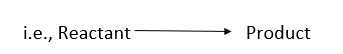The following are the general characters of a chemical change:
↪ A chemical change is a permanent change.
↪ A chemical change is accompanied either by energy evolution or absorption.
↪ A chemical change is always conserved un mass.

⁕ Differences between Physical and Chemical Change

 Physical Change Chemical Change 1. It is temporary change and process can be easily reversed. 1. It is permanent and irreversible change. 2. No new compound is formed, only the physical state and color may be changed. 2. New compound is formed with entirely different properties. 3. No change of mass. 3. Mass is always conserved. 4. Energy change is very small. 4. Energy change is large.

⁕ Chemical Equation

↪ A symbolic representation of a chemical change is called chemical equation.
↪ Reactants and products are denoted by their formulae.
↪ A chemical change is always conserved in mass, total number of atoms of reactants and products must be balanced in the given chemical equation.
↪ A balanced chemical equation is also called as stoichiometric equation.
Suppose a chemical change is given with word equation as: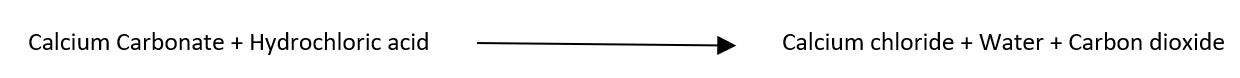and the chemical equation of the reaction is: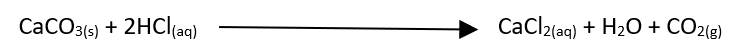⁕ Significance of a Chemical Equation

﻿Qualitative Significance

Consider a chemical equation of photosynthesis reaction:﻿↪ The given equation tells that carbon dioxide gas and water vapor undergoes photosynthesis reaction to give glucose and oxygen gas.
↪ Hence one can identify reactant and products with their physical states.

Quantitative Significance

Consider the equation: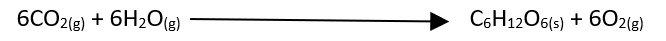The equation tells that:
6 molecules of CO2 + 6 molecules of H2O gives 1 molecule of C6H12O6 + 6 molecules of O2
or, 6 mol of CO2 + 6 mol of H2O gives 1 mol of C6H12O6 + 6 mol of O2
↪ Molecular mass of a substance expressed in gram is one mole or g. mole
6x44 g of CO2 + 6x18 g of H2O give 180 g of C6H12O6 + 192 g of O2
↪ Hence a chemical equation has its quantitative significance.

⁕ Limitations of Chemical Equation

↪ A chemical  equation does not give the concentration of reactants at the start and concentration of product and the end.
↪ It does not give rate of reaction and the time required for the completion of the reaction.
↪ It does not gives conditions required to carry out chemical reaction.
↪ A chemical equation does not give whether the heat is absorbed or evolved in the reaction.

⁕ Removal of defects

﻿In order to make chemical equation more informative the following corrections can be done:
↪ Physical states of components involved in the reaction are denoted by the symbols s, l, g and aq for solid, liquid, gas and aqueous respectively in the equation as: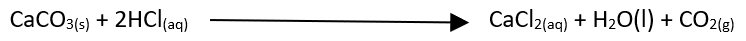↪ Reversibility of reaction is denoted by the notation ⇌ in the chemical equation.
↪ Exothermicity and endothermicity are mentioned by notation ∆H = -ve for exothermic reaction and ∆H = +ve for endothermic reaction at product side.
↪ External conditions can be given above and below the arrow indicating chemical equation.